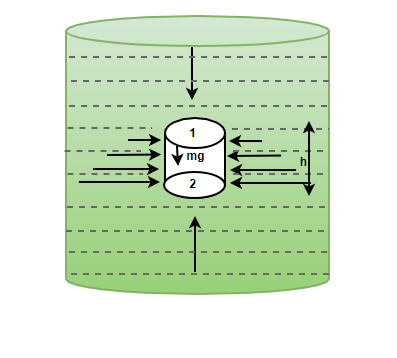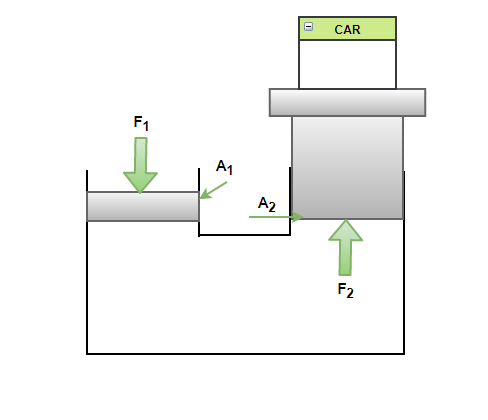GFG App
Open AppBrowser
Continue

Hydraulic machines are used in a lot of mechanical devices, from our cars to the springs used in trains. Hydraulic systems are involved. These systems are based upon a very basic principle but are very effective. These systems can be used to lift very heavy weights which will otherwise be impossible to lift. Hydraulic machines are based on pressure. It is essential to understand these systems to get an overview of complex machinery that is used all around us. Let’s look at this concept in detail.

### Pascal’s Law

French scientist Blaise Pascal observed that pressure in a fluid at rest is the same at all points if these points are at the same depth. The figure below shows a cylindrical element in the fluid of area A and height h. Let the pressure at the top of the element be P1 and P2 at the bottom. Let us assume that the weight of the liquid is “mg” in this element. Then the difference in pressures between the two points is given by,

P1 – P2 = mgh/ANow, assuming the density of the liquid to be “d”, then the mass of the liquid in the element will be,

m = d(A.h)

⇒ m = d.A.h

Plugging this value of m in the above equation for the pressure difference,

P1 – P2 = dgh

This extra pressure with the height “h” is called gauge pressure.

### Hydraulic Machines

Let’s see what happens when we change the pressure on the liquid. According to Pascal’s law of transmission, whenever external pressure is applied to any part of a liquid. This pressure gets distributed in all directions equally. A number of devices are based on this principle. The hydraulic lift is also an application of this law. Consider a hydraulic lift as given in the figure below. The two pistons are separated by a space filled with liquid. A piston of small cross-section A1 is used to exert a force F1 directly on the liquid.The pressure that is exerted in the column is given by, P =. This is transmitted throughout the liquid, which results in the pressure being applied on the other piston. The area of the other piston is A2, the force felt by this piston is given by,⇒⇒Notice that the applied force is increased by the factor of. This property helps in hydraulic systems for lifting very heavyweights.

### Sample Problems

Question 1: Hydraulic Machines work on the application of:

1. Pascal’s Law
2. Newton’s Law
3. Law of Gravity
4. None of These

Hydraulic Machines are based on the Pascal’s law of transmission. It says,

Whenever external pressure is applied to any part of a liquid. This pressure gets distributed in all directions equally.

Question 2: Find the pressure difference that comes when someone goes 10m deep inside the water. Given, the density of water = 1000Kg/m3

The difference between the pressures is given by,

P1 – P2 = dgh

Given: d = 1000, g = 10 and h = 10

P1 – P2 = dgh

⇒P1 – P2 = (1000)(10)(10)

⇒P1 – P2 = 105 Kg/m2

Question 3: Find the pressure difference that comes when someone goes 50m deep inside a liquid. Given the density of water = 500Kg/m3

The difference between the pressures is given by,

P1 – P2 = dgh

Given: d = 500, g = 10 and h = 50

P1 – P2 = dgh

⇒P1 – P2 = (500)(10)(50)

⇒P1 – P2 = 25 × 103 Kg/m2

Question 4: A hydraulic piston has two ends of the area A1 = 1m2 and A2 = 0.2m2. A Force of 100N is applied on the piston with a smaller area. Find the force on the other end.

In a hydraulic system, the force on the other end is given by,Given: A1 = 1m2 and A2 = 0.2m2. F1 = 100N

Plugging the values in the equation,⇒⇒Question 5: A hydraulic piston has two ends of the area A1 = 0.5m2 and A2 = 0.1m2. A Force of 50N is applied on the piston with a smaller area. Find the force on the other end.

In a hydraulic system, the force on the other end is given by,Given: A1 = 0.1m2 and A2 = 0.5m2. F1 = 50N

Plugging the values into the equation,⇒⇒Question 6: A hydraulic piston has two ends of the area A1 = 0.4m2 and A2 = 0.1m2. The goal is to pick the 50Kg box kept on the piston with 0.4 m2 area. , find the force that should be applied at the other end.

In a hydraulic system, the force on the other end is given by,Given: A1 = 0.1m2 and A2 = 0.5m2. F2 = 500N

Plugging the values into the equation,⇒⇒My Personal Notes arrow_drop_up
Related Tutorials